# 教育プログラム

## 国際インターンシップ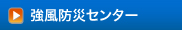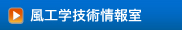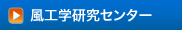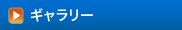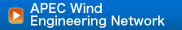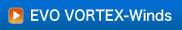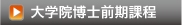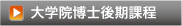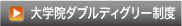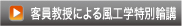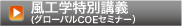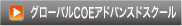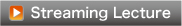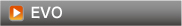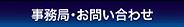グローバルCOE支援室
〒243-0297

TEL＆FAX046-242-9658（直通）
E-mail:gcoeoffice@arch.t-kougei.ac.jp

 国際インターンシップ講義　現在このプログラムは行なわれていません。
 国際インターンシップ学生・グローバルＣＯＥ短期研修員を対象に、大学院建築学・風工学専攻教授による風工学に関する集中講義を実施している。 【2008年度の講義内容】 【構造系分野】 1. Prof. Yukio Tamura Lecture 1: Introduction of TPU Global Center of Excellence Program Lecture 2: Risk under earthquakes and risk under wind Lecture 3: Extreme wind events and damage assessment Lecture 4: Flow patterns and pressures Lecture 5: Dynamic wind forces and design wind loads Lecture 6: Wind resistant design of tall buildings Lecture 7: Wind tunnel tests and full-scale tests Lecture 8: Monitoring techniques in wind engineering Lecture 9: Most efficient observation of random phenomena - Proper Orthogonal Decomposition Lecture 10: Damping in buildings Lecture 11: Suppression of wind-induced vibration of buildings - Damping devices Lecture 12: Pressure distributions and flow fields causing extreme wind force components Lecture 13: Universal equivalent static wind load distributions Lecture 14: Wind-induced nonelastic response 【環境系分野】 1. Prof. Ryuichiro Yoshie Numerical simulation of fluid flow and heat transfer Lecture 1. Equation of heat conduction ・Let’s check dimension (unit) of each term in the equation of heat conduction, and imagine physical meaning of the equation. ・Let’s derive the equation of heat conduction. ・Various expressions for the equation of heat conduction. Lecture 2. Numerical simulation for equation of heat conduction. ・Discretization by control volume method ・Discretization by finite difference ・Boundary conditions for heat transfer ・Explicit method ・Implicit method ・Numerical stability of explicit and implicit method ・Let’s make a numerical analysis program for the equation of heat conduction. Lecture 3. Equation of fluid flow and heat transfer ・Heat transport equation. ・Mass conservation equation (Continuity equation) ・Navier-Stokes equations ・Turbulence models ・Reynolds average ・Zero-equation model ・One-equation model ・Two-equation k-εmodel Lecture 4. Numerical simulation for Two-equation k-εmodel ・Discretization by control volume method ・MAC method ・SIMPLE method ・Four basic rules for discretization ・Upwind-scheme for advection term ・Boundary conditions ・Inflow boundary conditions ・Outflow boundary conditions ・boundary conditions for wall shear stress ・boundary conditions for wall heat transfer 2. Prof. Nobuyuki Kobayashi Assessment of Wind Environment for Pedestrian in Urban Area Lecture 1　Reality of Environmental obstacle by strong wind ・Assessment code of Tokyo Metropolitan Government ・Significance of “windy place” and “calm place” Lecture 2　Statistics of meteorological wind data ・Wind rose and wind speed ・Weibull distribution ・Gust factor of wind near the ground ・Wind tunnel test Approaching flow／Roughness elements／Reference wind speed in the wind tunnel／Wind speed ratio of each wind direction Lecture 2　Probability of exceedance of wind speed ・Application of meteorological wind data for exceeding probability ・Criteria for wind environment assessment by several researchers 3. Prof. Masaaki Ohba Natural ventilation and Cross- ventilation Lecture 1: Physics of natural ventilation Lecture 2: Cross-ventilation structure Lecture 3: Effective method of cross-ventilation Lecture 4: Energy-saving strategy and architectural design about cross-ventilation Lecture 5: Thermal comfort in cross-ventilation environment Indoor air pollution Lecture 1: Air pollution status caused by indoor chemical substances in houses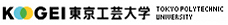サイトポリシー Copyright(c) TOKYO POLYTECHNIC UNIVERSITY All rights reserved ▲PAGE TOP